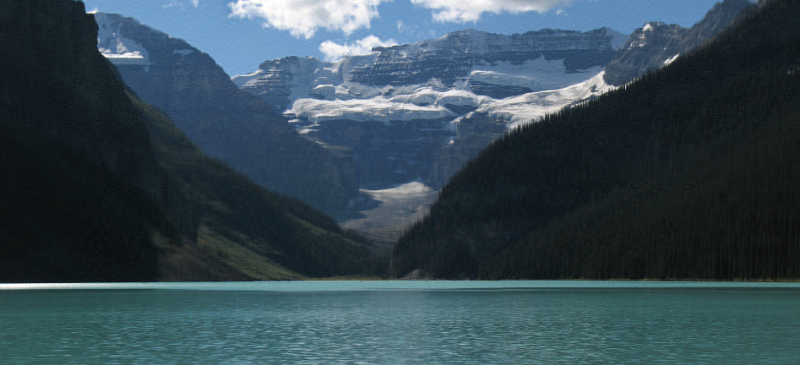## SVD applied to image compression

### Original image, 365x800 (=292,000 pixels)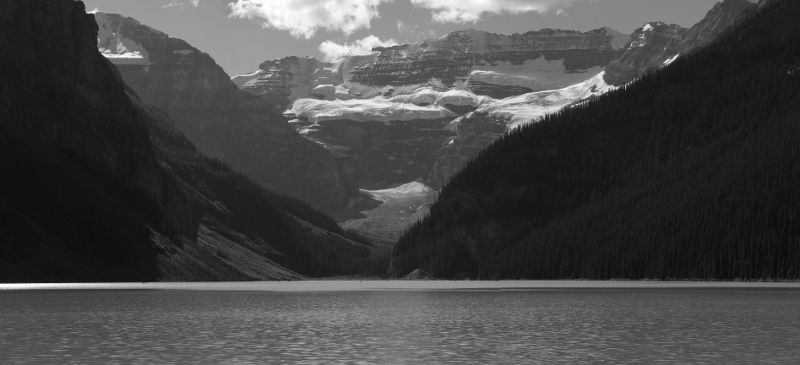### Rank k approximation, k=1 (requires 1166 numbers, or 0.4% of original)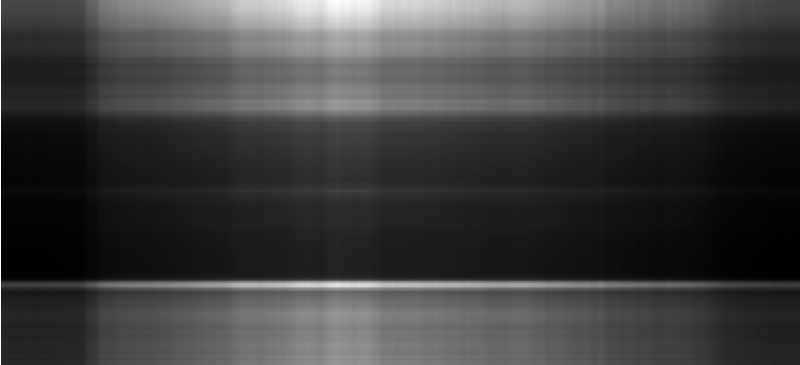### Rank k approximation, k=5 (requires 5830 numbers, or 2% of original)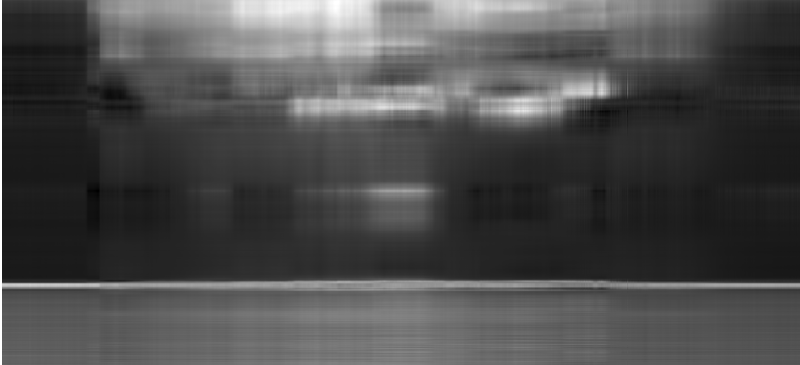### Rank k approximation, k=20 (requires 23,320 numbers, or 8% of original)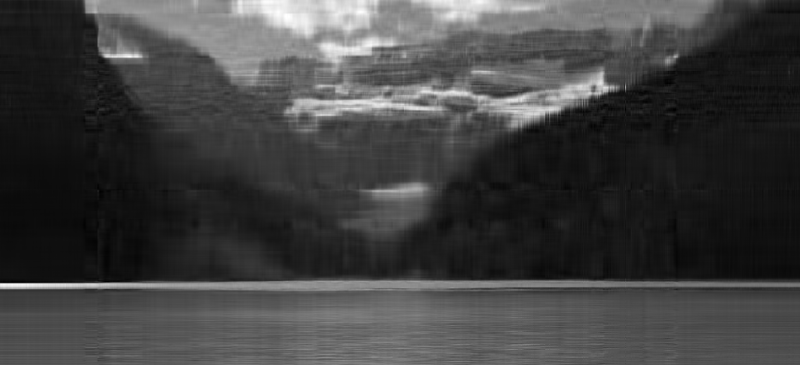### Rank k approximation, k=50 (requires 58,300 numbers, or 20% of original)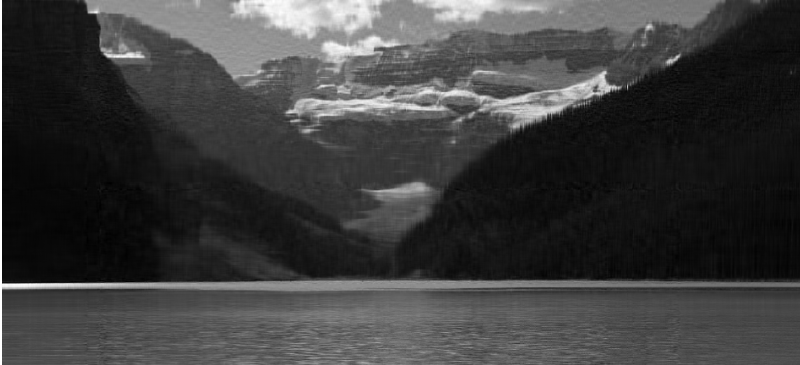### Rank k approximation, k=100 (requires 116,600 numbers, or 40% of original)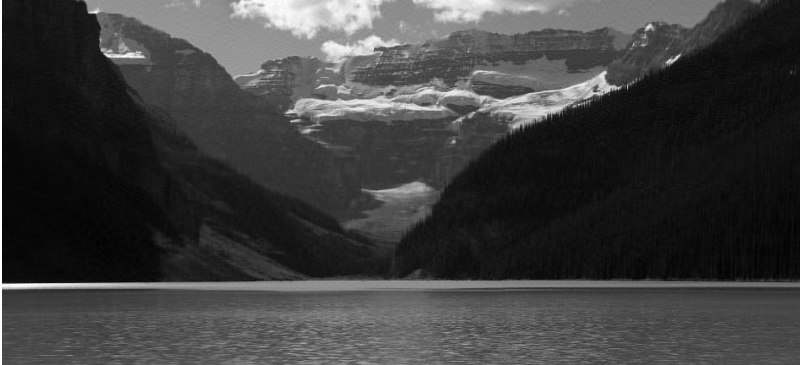### Original color image, 365x800, 3 color channels (=876,000 numbers)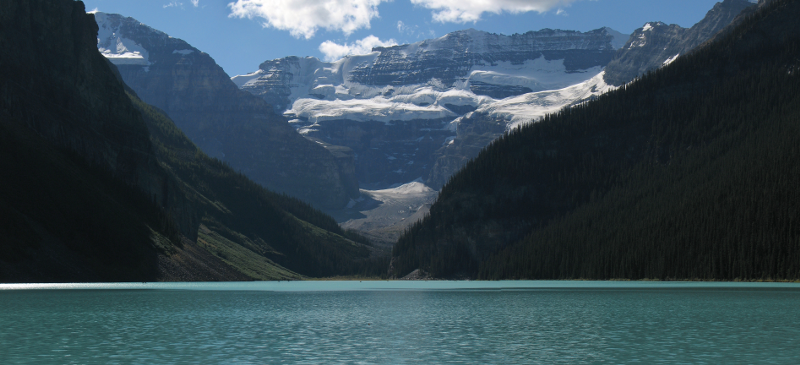### Rank k approximation, k=1 (requires 3498 numbers, or 0.4% of original)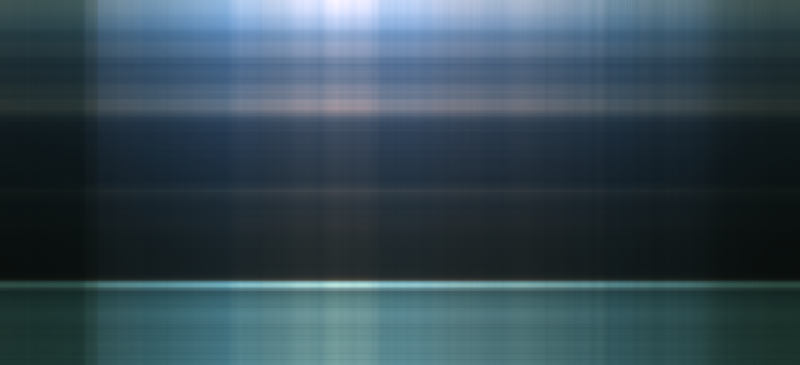### Rank k approximation, k=10 (requires 34,980 numbers, or 4% of original)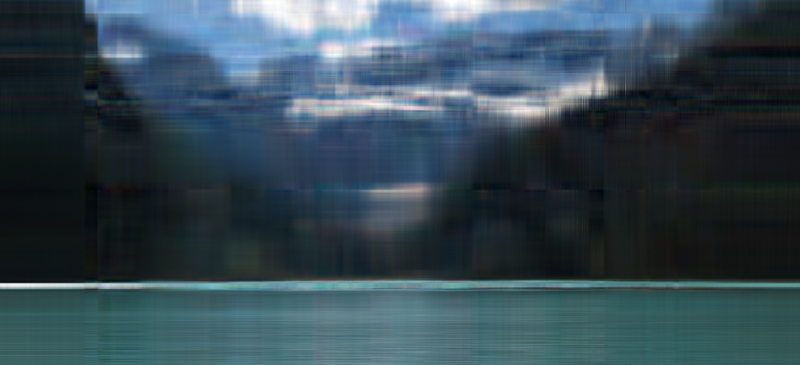### Rank k approximation, k=25 (requires 87,450 numbers, or 10% of original)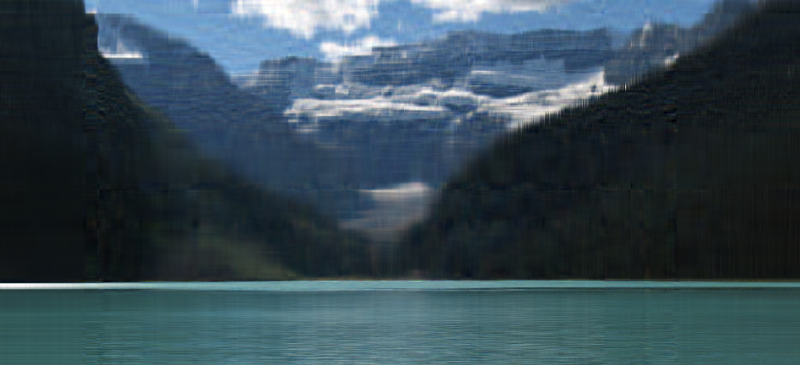### Rank k approximation, k=50 (requires 174,900 numbers, or 20% of original)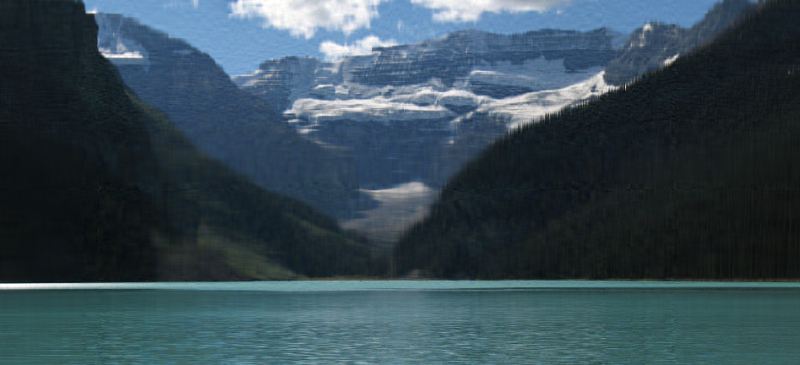### Rank k approximation, k=100 (requires 349,800 numbers, or 40% of original)